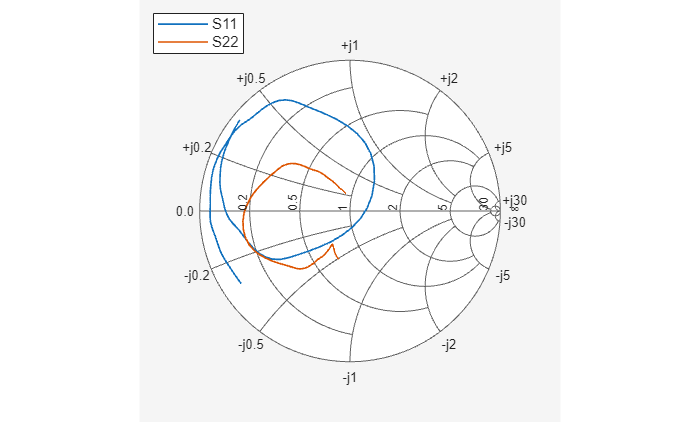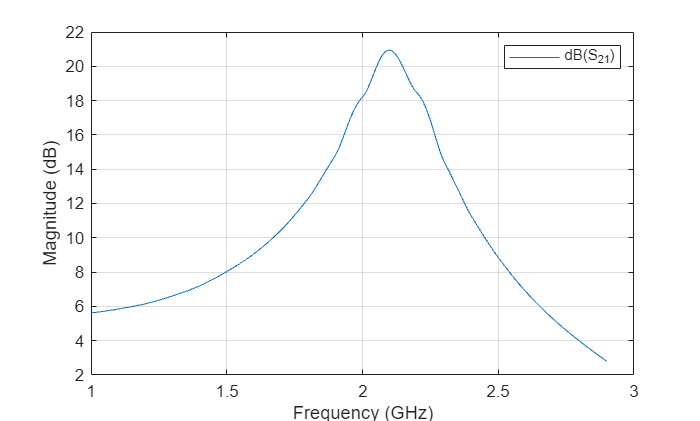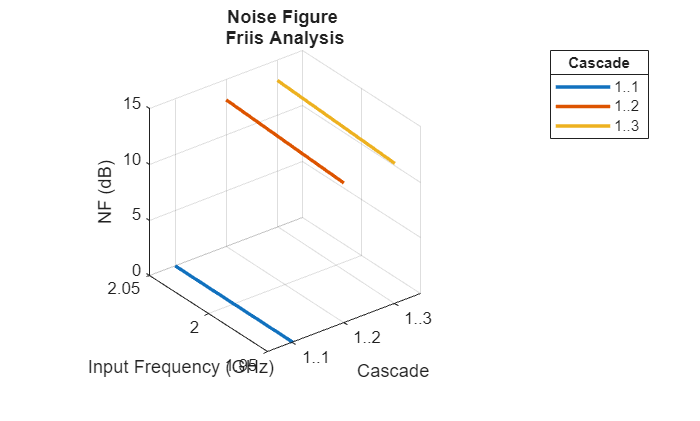This example shows you how to model a cascaded network, analyze the network in the frequency domain, and plot the results. The network that you use in this example consists of an amplifier and two transmission lines.

#### Create RF Components

Create three circuit (`rfckt`) objects with the default property values. These circuit objects represent the two transmission lines and the amplifier.

```FirstCkt = rfckt.txline; SecondCkt = rfckt.amplifier; ThirdCkt = rfckt.txline;```

#### Specify Component Data

In this part of the example, you specify the transmission line and amplifier properties.

Transmission Line Properties

Set the line length of the first transmission line, `FirstCkt`, to `12`.

`FirstCkt.LineLength = 12;`

Set the line length of the second transmission line, `ThirdCkt`, to `0.025` and the phase velocity to `2.0e8`.

```ThirdCkt.LineLength = 0.025; ThirdCkt.PV = 2.0e8;```

Amplifier Properties

Import network parameters, noise data, and power data from the `default.amp` file into the amplifier, `SecondCkt`.

`read(SecondCkt,'default.amp');`

Set the interpolation method of the amplifier, `SecondCkt`, to `cubic`.

`SecondCkt.IntpType = 'cubic';`

The `IntpType` property tells the RF toolbox how to interpolate the network parameters, noise data, and power data when you analyze the amplifier at frequencies other than those specified in the file.

#### Validate RF Components

In this part of the example, you plot the network parameters and power data (output power versus input power) to validate the behavior of the amplifier. Use the `smithplot` function to plot the original S11 and S22 parameters of the amplifier (`SecondCkt`) on a Z Smith® Chart.

```figure legend show lineseries1 = smith(SecondCkt,'S11','S22'); lineseries1(1).LineStyle = '-'; lineseries1(1).LineWidth = 1; lineseries1(2).LineStyle = ':'; lineseries1(2).LineWidth = 1;```Plot the amplifier (`SecondCkt`) output power (Pout) as a function of input power (Pin), both in decibels referenced to one milliwatt (dBm), on an X-Y plane plot.

```figure legend show plot(SecondCkt,'Pout','dBm')```#### Build and Simulate Network

In this part of the example, you create a circuit object to represent the cascaded amplifier and analyze the object in the frequency domain. Cascade the three circuit objects to form a new cascaded circuit object, `CascadedCkt`.

```FirstCkt = rfckt.txline; SecondCkt = rfckt.amplifier; ThirdCkt = rfckt.txline; CascadedCkt = rfckt.cascade('Ckts',{FirstCkt,SecondCkt,... ThirdCkt});```

Define the range of frequencies over which to analyze the cascaded circuit, and then run the analysis.

```f = (1.0e9:1e7:2.9e9); analyze(CascadedCkt,f);```

The plot shows the power data at 2.1 GHz because this frequency is the one for which power data is specified in the `default.amp` file.

#### Analyze Simulation Results

In this part of the example, you analyze the results of the simulation by plotting data for the circuit object that represents the cascaded amplifier network. Use the `smithplot` function to plot the S11 and S22 parameters of the cascaded amplifier network on a Z Smith Chart.

```figure legend show lineseries2 = smith(CascadedCkt,'S11','S22','z'); lineseries2(1).LineStyle ='-'; lineseries2(1).LineWidth =1; lineseries2(2).LineStyle = ':'; lineseries2(2).LineWidth = 1;```Use the `plot` function to plot the S21 parameter of the cascaded network, which represents the network gain, on an X-Y plane.

```figure legend show plot(CascadedCkt,'S21','dB')```Use the `plot` function to create a budget plot of the S21 parameter and the noise figure of the amplifier network:

```figure legend show plot(CascadedCkt,'budget', 'S21','NF')```The budget plot shows parameters as a function of frequency by circuit index. Components are indexed based on their position in the network. In this example:

• Circuit index one corresponds to `FirstCkt`.

• Circuit index two corresponds to `SecondCkt`.

• Circuit index three corresponds to `ThirdCkt`.

The curve for each index represents the contributions of the RF components up to and including the component at that index.